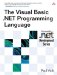# Comparison Operators

 <  Day Day Up  >

The comparison operators are used to compare two values. They take two operands of any type and result in a Boolean value as to whether the particular comparison is true or false. The comparison operators are listed in Table 5-3.

The Is and = operators each test for equality, but the Is operator tests reference equality, while the = operator tests value equality. Is can only be used on two references and determines whether the two references refer to the same location on the heap. The = operator, on the other hand, can only be used on the fundamental types and determines only whether the two values are the same, not whether they are stored at the same location. For example:

##### Table 5-3. Comparison Operators

Operators

Description

Is

Reference equality

=

Equality

<>

Inequality

<

Less than

>

Greater than

<=

Less than or equal to

>=

Greater than or equal to

` Class cl End Class Module Test   Sub Main()     Dim x, y As Integer     Dim a, b, c As cl     a = New cl()     b = a     c = New cl()     If a Is b Then       Console.WriteLine("a and b are the same instance of cl.")     End If     If a Is c Then       Console.WriteLine("a and c are the same instance of cl.")     End If     x = 5     y = 5     If x = y Then       Console.WriteLine("x and y are equal.")     End If   End Sub End Module `

This example will print " a and b are the same instance of cl. " because a and b each hold a reference to the same instance. Similarly, the example will print " x and y are equal. " because they each hold the same value. (Reference types are covered in more detail in Chapter 9, Classes and Structures.)

When String values are compared, comparisons can be done either as text comparisons or as binary comparisons . A text comparison compares two strings using the culture of the current thread at runtime. The culture is used to compare each character and determine whether the two characters are the same according to the current culture. Thus, when a text comparison is done, it is possible that two characters with different Unicode values may nonetheless compare as equal if they are considered to be the same in the current culture. On the other hand, a binary comparison compares two strings according to whether each character has the same Unicode code-point value. Binary comparisons will return the same value regardless of the culture of the current thread.

The property Thread.CurrentThread can be used to get an object that represents the current thread of execution. The CurrentCulture property on the object can be used to get and set the current culture being used.

By default, string comparisons are done as binary comparisons. An Option Compare statement at the beginning of the file can change the default for that file. Option Compare Binary specifies that binary comparisons are to be used in the file, while Option Compare Text specifies text comparisons for the file.

## Style

Text comparisons are significantly slower than binary comparisons because they require looking up information about the current culture. Unless culture-sensitive comparisons are needed, binary comparisons are recommended.

 <  Day Day Up  >The Visual Basic .NET Programming Language
ISBN: 0321169514
EAN: 2147483647
Year: 2004
Pages: 173
Authors: Paul Vick

Similar book on Amazon